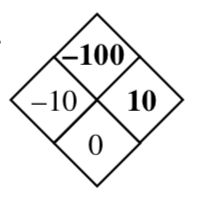### Home > CCA > Chapter 1 > Lesson 1.2.1 > Problem1-33

1-33.

Copy these Diamond Problems and use the pattern you discovered earlier, shown at right, to complete each of them. Some of these may be challenging! Homework Help ✎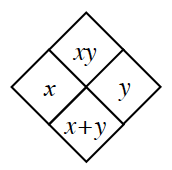1.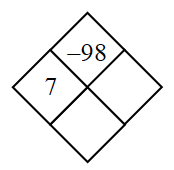Look at the pattern. You have $xy$ and $x$. How can you use those to fill in the rest of the diamond?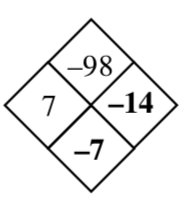1.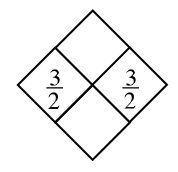To find the top answer, multiply the two given numbers. To find the bottom answer add the two given numbers.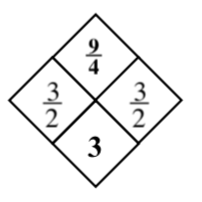1.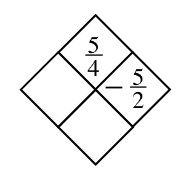Refer to part (a).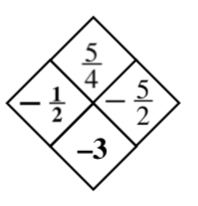1.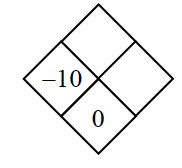Refer to part (a).